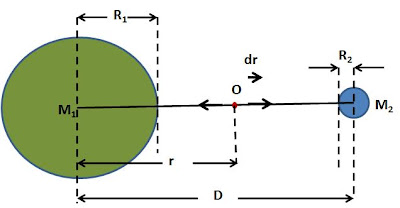## Monday, November 10, 2008

### Irodov Problem 1.232Let the masses of the Earth and Moon be M1 and M2 and let their radii be R1 and R2. Let the distance between the center of Earth and Moon be D. The total force acting on the spaceship when it is at a distance r from the center of the Earth is given by,The work done by the spaceship (which is in the opposite direction to the gravitational force acting on the spaceship and hence the negative sign) is given by,Now if the distance D is considered much much larger than R1 or R2 then, the work done is given by,